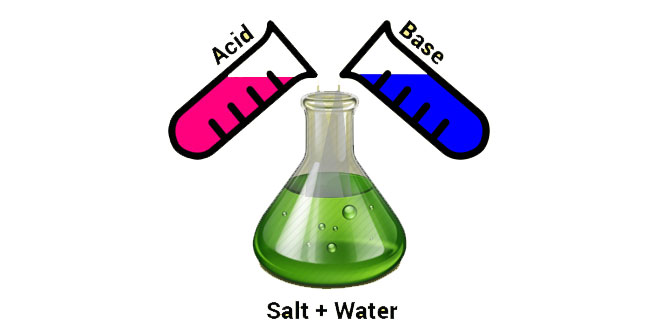Wednesday , September 28 2022# Acids, Bases and Salts: 10th Science

#### Question: What is meant by p and pH?

Answer: p in pH stands for ‘potenz’ in Germany and H is for hydrogen.

#### Question: What are antacids? Explain their role in providing relief from stomach ache.

1. Antacids are mild base.
2. Antacids neutralise the effect of extra acid produced in the stomach during indigestion and thus provide relief.

#### Question: How does the change occur in values of milk when it changes to curd?

Answer: When milk changes to curd, it becomes acidic due to the formation of lactic acid. Thus, its pH value decreases and becomes less than 6.

#### Question: Define alkalies and give an example.

Answer: The bases soluble in water are called alkalies. e.g., NaOH, KOH.

#### Question: Mention the range of pH value for a identification of a base.

Answer: pH value for identification of a base is 7-14.

#### Question: How chloride of lime differs chemically from calcium chloride?

Answer: Chloride of lime is CaOCl2 while calcium chloride is CaCl2.

#### Question: What is meant by “water of crystallization” in a substance? Explain with an example.

Answer: Water of crystallization the fixed number of water molecules present in one formula unit of a salt.
Example: Hydrated copper sulphate (CuSO4.5H2O). Here, 5H2O signify five molecules of water of crystallization.

#### Question: Write the chemical name and chemical formula of washing soda.

Answer: Chemical name: Sodium carbonate
Chemical formula: Na2CO3.10H2O

#### Question: What effect does an increase in concentration of H+(aq) in a solution have on the pH of solution?

Answer: The pH of a solution decrease with the increase in the concentration of H+(aq) in a solution. More are the H+(aq) in a solution, more is the acidity, and less is the pH value of that solution.

#### Question: Name the gas evolved when dilute HCl reacts with sodium hydrogen carbonate. How is it recognized?

Answer: The gas evolved is CO2. When this gas is passed through lime water, it turns it milky.

#### Question: What are olfactory indicators?

Answer: The substances whose odour changes in acidic or basic media are called olfactory indicators.

#### Question: Why does 1 MHCl solution have a high concentration of H+ ions than 1 M CH3COOH solution?

Answer: HCl solution is a strong acid and, therefore, ionises completely as compared to CH3COOH solution which is a weak acid. Due to complete ionisation, it contains high concentration of Hions. 2

#### Question: Which one of the following has a higher concentration of  H+ ions? 1 M HCl or 1 M CH3COOH.

Answer: 1 M HCl has higher concentration of Hions. Being strong acid, it ionises completely to furnish Hions.

#### Question: How will you test for the gas which is liberated when hydrochloric acid reacts with an active metal?

Answer: When burning candle is brought near to the jar filled with hydrogen gas, it burns explosively with a pop sound.

#### Question: In which form H+(hydrogen) ions exist in water?

Answer: Since hydrogen ions cannot exist alone, therefore, they exist after combining with water molecules. Thus, hydrogen ions must be shown as H(aq) or hydronium ion (H3O+).

#### Question: How is the pH of a solution of an acid influence when it is diluted?

Answer: On dilution, the concentration of hydrogen ions per unit volume decreases and hence pH of the solution increases. Since pH is the negative logarithmic value of the concentration of hydrogen ions.

#### Question: At what pH rain water is said to be acidic?

Answer: When pH of rain water is less than 5.6, then it is called acid rain.

#### Question: Which gas is evolved when dilute hydrochloric acid reacts with zinc? Write the molecular formula of this gas.

Answer: Hydrogen gas. Molecular formula is H.

#### Question: Dry HCl gas does not change the colour of dry litmus. Give reason.

Answer: Hion from HCl cannot ionise to give Hions in absence of water. Acidic property like change in colour of litmus depends on production of Hion, hence there is no colour change.

#### Question: Why is HCl a stronger acid than acetic acid?

Answer: On dissociation, it yields larger [H+] Hydrogen ions for the same concentration as compared to acetic acid.

#### Question: Tooth enamel is the hardest substance in our body. Name the compound of which it is made-up of. At what pH of the mouth it gets corroded? State the role of bacteria present in the mouth. Suggests a method to present tooth decay.

Answer: Calcium phosphate; below 5.5; bacteria produce acids by degradation of sugar and food particles remaining in the mouth after eating; using tooth pastes which are generally basic.

## Sources of Energy: 10th Science Chapter 14

Class: 10th Class Subject: Science Chapter: Chapter 14: Sources of Energy Quiz: – Questions MCQs: …